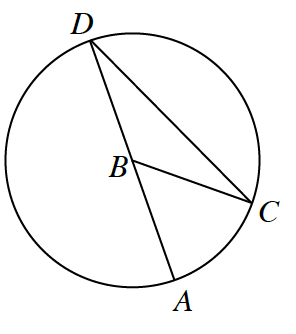### Home > GC > Chapter 10 > Lesson 10.1.3 > Problem10-28

10-28.

Assume point $B$ is the center of the circle below. Match each item in the left column with the best description for it in the right column.

1. $\overline{AB}$

1. inscribed angle

1. $\overline{CD}$

1. semicircle

1. $\overarc { A D }$

1. $∠CDA$

1. minor arc

1. $\overarc{ A C }$

1. central angle

1. $∠ABC$

1. chordRefer to the glossary in your textbook for a definition of each description.

(a) matches to ($3$)

(b) matches to ($6$)